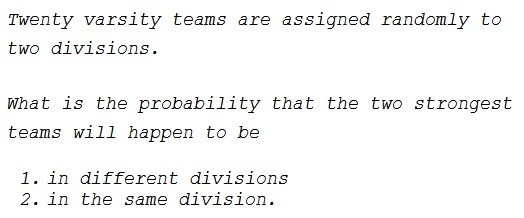# Two Varsity Divisions

### Problem### Solution 1

Twenty teams may be split into two divisions of $10$ teams each in $\displaystyle \frac{1}{2}{20\choose 10}$ ways. Two teams removed, $18$ to be split between the two divisions. For the first question, we need $9$ teams to complete the division with one of the strongest teams (the other division will be filled automatically.) Thus the probability in Question 1 is

$\displaystyle P=\frac{18\choose 9}}\frac{1}{2}{20\choose 10}}=\frac{10}{19}$

For the second question, we need $8$ teams to complete the division of the two strongest teams. Thus the probability in Question 2 is

$\displaystyle P=\frac{18\choose 8}}\frac{1}{2}{20\choose 10}}=\frac{9}{19}$

### Solution 2

Denote, for convenience, the two strongest teams $A$ and $B.$ When $A$ is chosen into one of the divisions, there are $19$ slots that $B$ can fit in. For the first question, $10$ of these slots are in the "other" division, giving the probability as $\displaystyle \frac{10}{19}.$

For the second question, there are $9$ slots in the same division as $A,$ making the probability of $B$ falling there equal to $\displaystyle \frac{9}{19}.$

Naturally, if $A$ and $B$ are not in the same division, then they are in different ones, so that $\displaystyle \frac{10}{19}+\frac{9}{19}=1.$

### Acknowledgment

That's a modification of a problem from A. M. Yaglom, I. M. Yaglom, Challenging Mathematical Problems with Elementary Solutions, Vol I, Dover, 1987. But I have only a Russian edition.